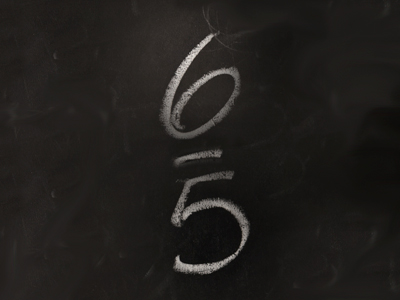65 is an improper fraction, equal to the mixed fraction 1 15.

# Fractions 2 (Difficult)

If you’ve been playing all our Eleven Plus maths quizzes in order, you’ll know by now that fractions are very important. But you will also be very confident when dealing with them, won’t you?

You should, by now, know the difference between a numerator and a denominator and be familiar with improper and mixed fractions. You should also be able to add and subtract fractions, and use them to work out proportions as fractions.

With all that experience under your belt, even though it’s difficult, this quiz should pose you no problems. Just make sure you read the questions carefully and consider them a while before you choose your answers. You might also like to read the helpful comments after each question – they clarify each point, making sure you understand.

And now, if you are ready, it’s time to begin. Good luck!

1.
What is 266 as a mixed fraction in its simplest form?
4 12
4 13
4 14
4 16
To convert an improper fraction to a mixed fraction, follow these steps: 1. Divide the numerator by the denominator. 2. Note the whole number remainder. 3. Write the number from step 1 as the whole number in front of the fractional part AND write the fractional part with the remainder in the numerator and keep the original denominator. STEP 1: 26 ÷ 6 = 4. STEP 2: Remainder 2. STEP 3: 4 26. Divide the numerator and denominator by 2 to reduce the fraction to its simplest form: 4 13
2.
What is 14 810 as an improper fraction in its simplest form?
745
14810
1208
312
To convert a mixed fraction to an improper fraction, follow these steps: 1. Multiply the whole number part by the denominator. 2. Add this result to the numerator. 3. Write the fraction with step 2 in the numerator and keep the original denominator. STEP 1: 14 × 10 = 140. STEP 2: 140 + 8 = 148. STEP 3: 14810. Divide the numerator and denominator by 2 to reduce the fraction to its simplest form: 745
3.
How many 418 are there in 23?
6
4
2
3
418 can be reduced to 29 (divide the numerator and the denominator by 2) and 23 can be turned into 69 (multiply the numerator and the denominator by 3) . Now you can see that 69 is thrice as much as 29
4.
What is 5 416 as an improper fraction in its simplest form?
112
214
418
8416
To convert a mixed fraction to an improper fraction, follow these steps: 1. Multiply the whole number part by the denominator. 2. Add this result to the numerator. 3. Write the fraction with step 2 in the numerator and keep the original denominator. STEP 1: 5 × 16 = 80. STEP 2: 80 + 4 = 84. STEP 3: 8416. Divide the numerator and denominator by the same number, in this case 4, to reduce the fraction to its simplest form: 214
5.
What is 428 as a mixed fraction in its simplest form?
5 24
5 12
5 14
5 18
To convert an improper fraction to a mixed fraction, follow these steps: 1. Divide the numerator by the denominator. 2. Note the whole number remainder. 3. Write the number from step 1 as the whole number in front of the fractional part AND write the fractional part with the remainder in the numerator and keep the original denominator. STEP 1: 42 ÷ 8 = 5. STEP 2: Remainder 2. STEP 3: 5 28. Simplify the fraction by dividing the numerator and denominator by the same number, in this case 2, to get your final answer, 5 14.
6.
A 112 x 112 is the same as what fraction of a sixth?
112
136
124
148
112 x 112 = 1144. You now have to find a fraction which multiplied with 16 gives 1144. Well, 144 ÷ 6 = 24, so the fraction (in its simplest form) must be 124
7.
If 51100 of the electorate voted for the government, how many people voted against the government?
24 people
49 people
2450 of the electorate
49100 of the electorate
51100 of the electorate voted for the government BUT fractions alone DO NOT tell you the actual number of people involved. If you had been told that there were 100 voters, then choice 2 would have been the best answer. Always be on the lookout for the BEST answer
8.
What is 34 of 415 in its simplest form?
15
14
13
16
The word OF means multiply: 34 x 415 = 1260. You multiply the numbers in the denominator together, and you multiply the numbers in the numerator together to form a single fraction. This fraction can then be reduced to its simplest form: sometimes you can reduce the fractions before you multiply them together
9.
There are 28 pupils in a class. If 47 of them are girls, how many boys are in the class?
8
12
16
14
All you have to do is find 47 of 28 and subtract it from 28: 47 × 28 = (4 × 28) ÷ 7 = 112 ÷ 7 = 16. That's the number of girls. To find the number of boys, just subtract 16 from 28: 28 - 16 = 12
10.
What is 56 + 23 + 618 expressed as a mixed fraction?
1 13
1 23
1 56
1 1718
First, make all the fractions have the same denominator: 56 + 23 + 618 = 56 + 46 + 26. Here we see a shortcut: 46 + 26 = 1, so the answer is 1 56
Author:  Frank Evans